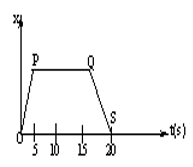The given Fig represents the displacement (X) time (t) graph for a particle in one dimensional motion Which of the fig given below represents the variation in velocity of the particle with time

# The given Fig represents the displacement (X) time (t) graph for a particle in one dimensional motion Which of the fig given below represents the variation in velocity of the particle with time1. A
2. B

3. C
4. D

Register to Get Free Mock Test and Study Material

+91

Verify OTP Code (required)

### Solution:

Is the correct v-t graph. This is because from t=0 to 5sec, displacement increases linearly with time, velocity is uniform. From t=5 sec to 15 sec, displacement is constant, velocity is zero. From t=15 sec to 20sec, displacement decreases linearly with time. Therefore, velocity is negative, but constantRegister to Get Free Mock Test and Study Material

+91

Verify OTP Code (required)